# (1 point) Find the general solution to y + 8y' + 41y = 0. Give your...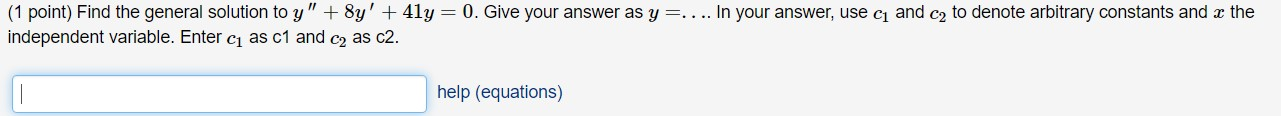(1 point) Find the general solution to y + 8y' + 41y = 0. Give your answer as y=.... In your answer, use ci and cz to denote arbitrary constants and the independent variable. Enter ci as c1 and ca as c2. help (equations)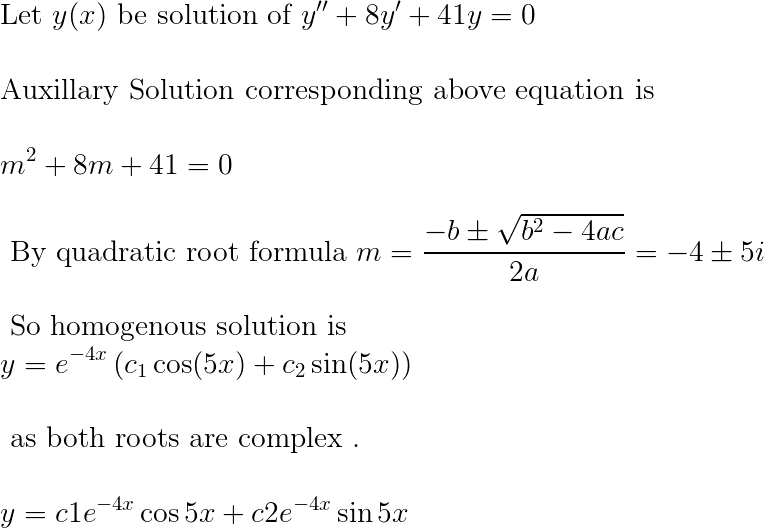##### Add Answer of: (1 point) Find the general solution to y + 8y' + 41y = 0. Give your...
Similar Homework Help Questions
• ### Consider the differential equation: y' - 5y = -2x – 4. a. Find the general solution...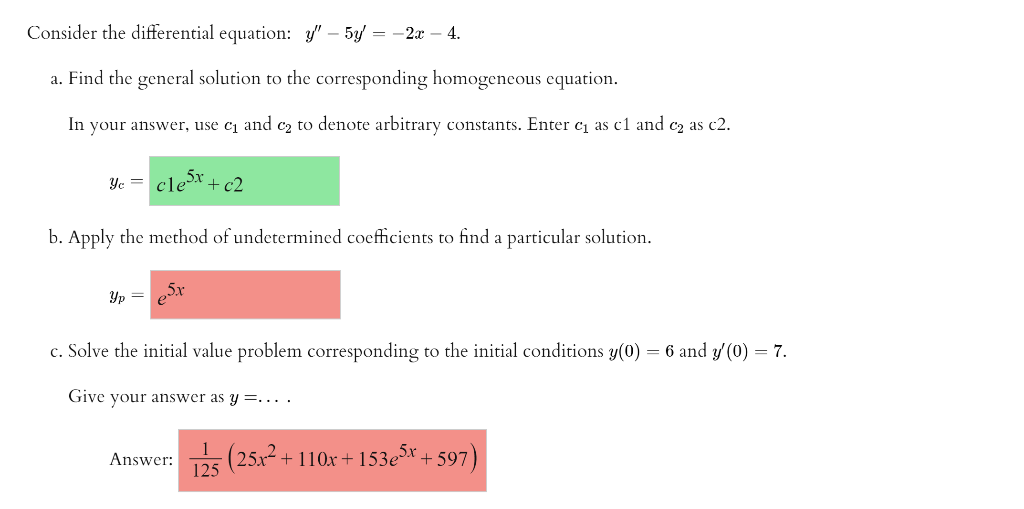Consider the differential equation: y' - 5y = -2x – 4. a. Find the general solution to the corresponding homogeneous equation. In your answer, use cı and ca to denote arbitrary constants. Enter ci as c1 and ca as c2. Yc = cle cle5x - + c2 b. Apply the method of undetermined coefficients to find a particular solution. yp er c. Solve the initial value problem corresponding to the initial conditions y(0) = 6 and y(0) = 7. Give...

• ### Section 3.4 Repeated Roots: Problem 1 Previous Problem Problem List Next Problem (1 point) Find the...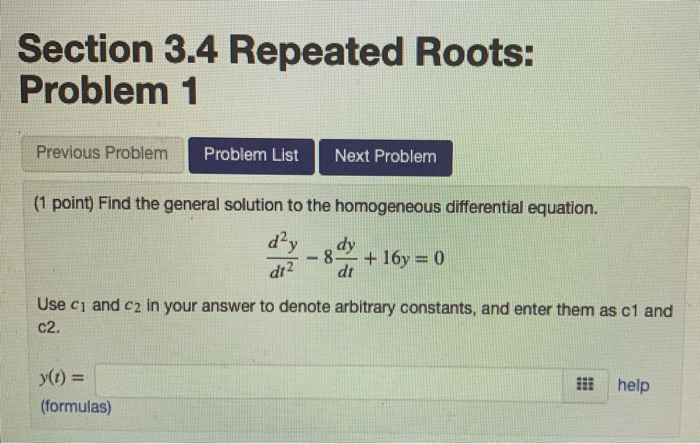Section 3.4 Repeated Roots: Problem 1 Previous Problem Problem List Next Problem (1 point) Find the general solution to the homogeneous differential equation. 2 dt dt Use ci and c2 in your answer to denote arbitrary constants, and enter them as c1 and C2. y(t) - (formulas) iii help

• ### Consider the differential equation: y" + 12y' + 36 = 6x2 + 5e-52. a. Find the...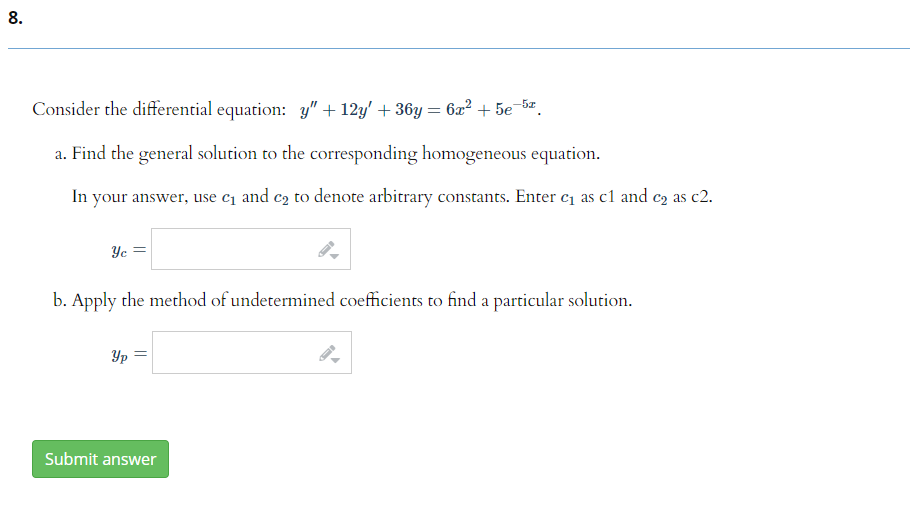Consider the differential equation: y" + 12y' + 36 = 6x2 + 5e-52. a. Find the general solution to the corresponding homogeneous equation. In your answer, use cy and ca to denote arbitrary constants. Enter C as c1 and ca as c2. Yc = b. Apply the method of undetermined coefficients to find a particular solution. Yp = Submit answer

• ### (1 point) For the differential equation y" + 4y' + 13y-0, a general solution is of...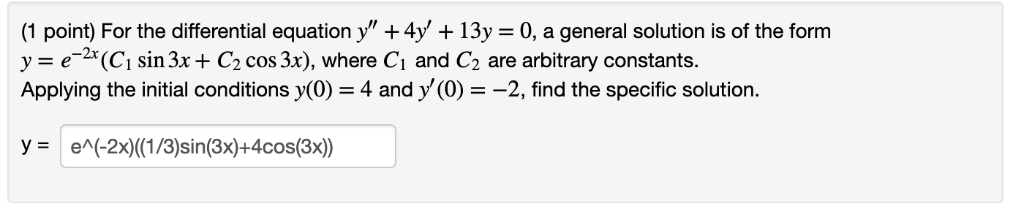(1 point) For the differential equation y" + 4y' + 13y-0, a general solution is of the form y(C sin 3xC2 cos 3x), where Ci and C2 are arbitrary constants. Applying the initial conditions y(0) 4 and y (0)-2, find the specific solution. y- en(-2x)((1/3)sin(3x)-4cos(3x))

• ### 5. Try again < Previous You have answered 1 out of 3 parts correctly. Consider the...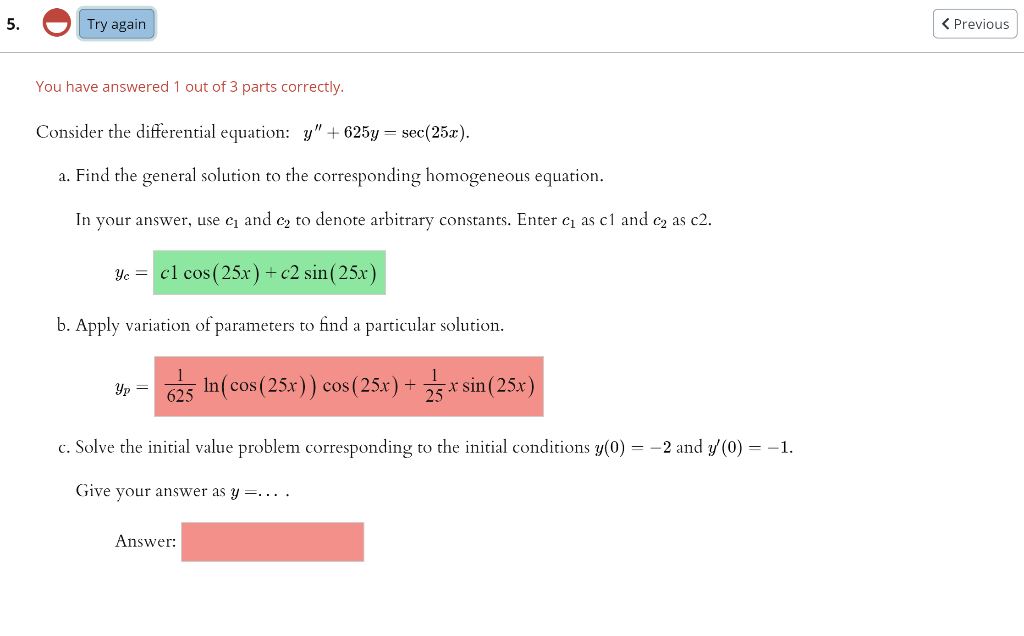5. Try again < Previous You have answered 1 out of 3 parts correctly. Consider the differential equation: y" +625y = sec (25x). a. Find the general solution to the corresponding homogeneous equation. In your answer, use ci and cy to denote arbitrary constants. Enter cı as c1 and ca as c2. Ye = cl cos(25x) + c2 sin (25x) b. Apply variation of parameters to find a particular solution. Yp = 625 In ( cos(25x)) cos(25x) + mars -x...

• ### (5 points) Suppose a spring with spring constant 6 N/m is horizontal and has one end...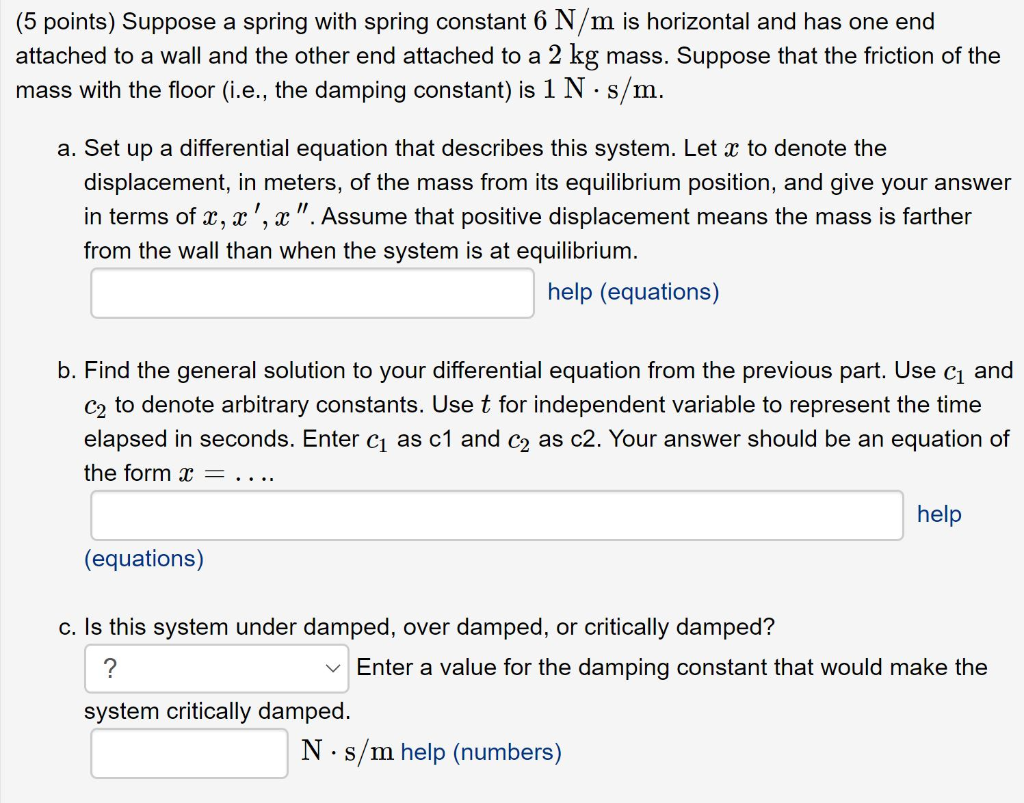(5 points) Suppose a spring with spring constant 6 N/m is horizontal and has one end attached to a wall and the other end attached to a 2 kg mass. Suppose that the friction of the mass with the floor (i.e., the damping constant) is 1 N.: ·s/m. a. Set up a differential equation that describes this system. Let x to denote the displacement, in meters, of the mass from its equilibrium position, and give your answer in terms of...

• ### please help The general solution of the equation y4y 0 is y = ccos(2x)c2sin(2x) Find values of ci and c2 so that y(0...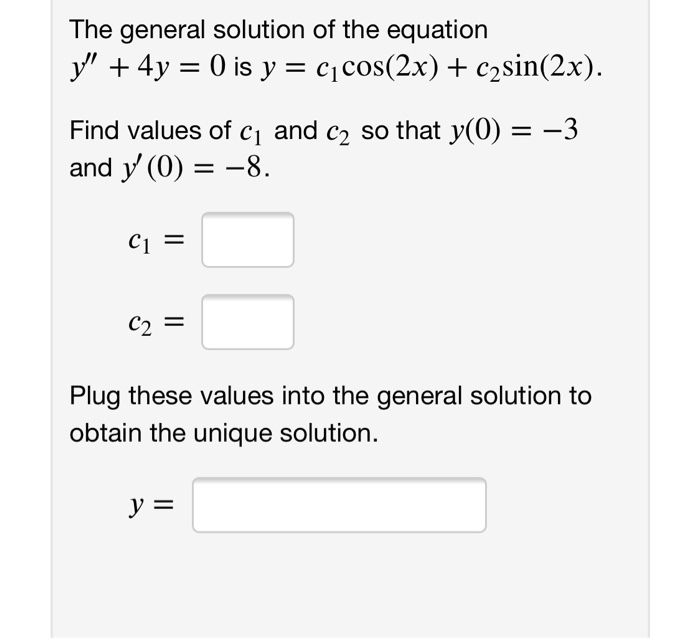please help The general solution of the equation y4y 0 is y = ccos(2x)c2sin(2x) Find values of ci and c2 so that y(0) and y (0) 8 -3 C1 = C2= Plug these values into the general solution to obtain the unique solution y = The general solution of the equation y4y 0 is y = ccos(2x)c2sin(2x) Find values of ci and c2 so that y(0) and y (0) 8 -3 C1 = C2= Plug these values into the general...

• ### (1 point) Use the indicated change of variable to find the general solution of the differential equation on (0, oo): General solution for w: w -cjJ +2J General solution for y: y- ci NOTE REGARDING AN...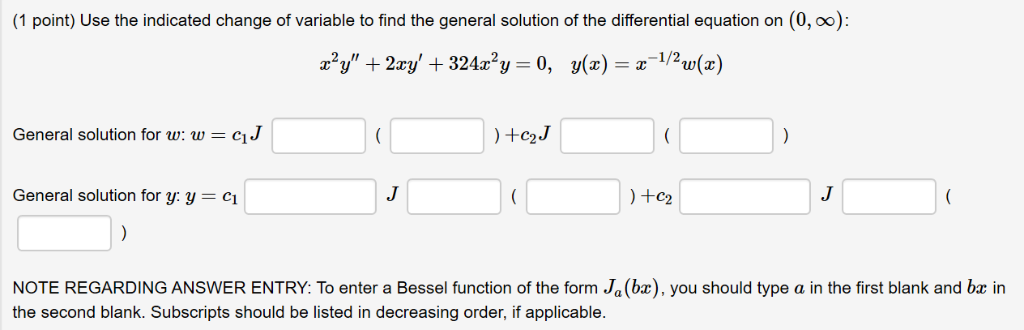(1 point) Use the indicated change of variable to find the general solution of the differential equation on (0, oo): General solution for w: w -cjJ +2J General solution for y: y- ci NOTE REGARDING ANSWER ENTRY: To enter a Bessel function of the form Ja(bx), you should type a in the first blank and bz in the second blank. Subscripts should be listed in decreasing order, if applicable. (1 point) Use the indicated change of variable to find the...

• ### Consider the ODE below. y' + 364 = sec(62) Find the general solution to the associated...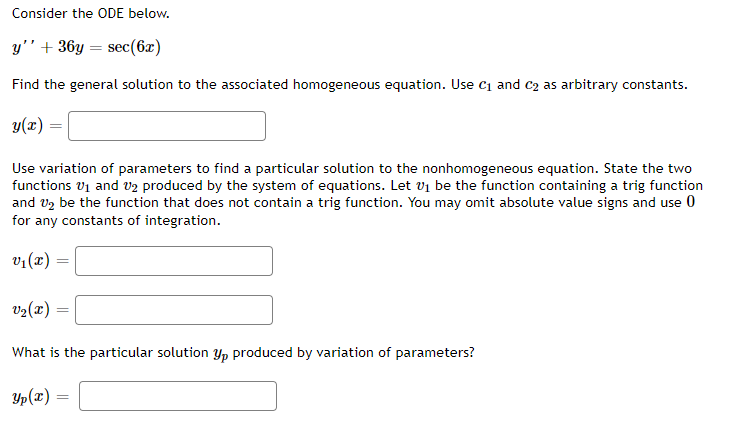Consider the ODE below. y' + 364 = sec(62) Find the general solution to the associated homogeneous equation. Use C1 and C2 as arbitrary constants. y(2) Use variation of parameters to find a particular solution to the nonhomogeneous equation. State the two functions vi and U2 produced by the system of equations. Let vi be the function containing a trig function and V, be the function that does not contain a trig function. You may omit absolute value signs and...

• ### 2 -e Recall that cosh(x) er te 2 and sinh(2) Any general solution of y'' –...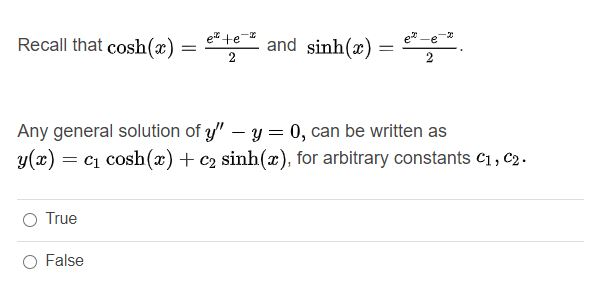2 -e Recall that cosh(x) er te 2 and sinh(2) Any general solution of y'' – y=0, can be written as y(x) = ci cosh(x) + C2 sinh(x), for arbitrary constants C1, C2. O True O False

Free Homework App